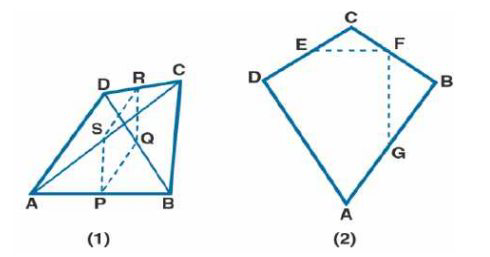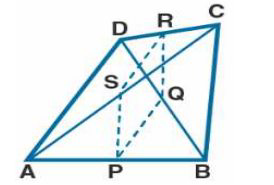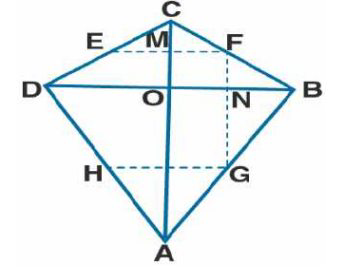# ML Aggarwal Solutions Class 9 Mathematics Solutions for Mid Point Theorem Exercise 11 in Chapter 11 - Mid Point Theorem

(a) In the quadrilateral (1) given below, AD = BC, P, Q, R, and S are mid-points of AB, BD, CD, and AC

respectively. Prove that PQRS is a rhombus.

(b) In the figure (2) given below, ABCD is a kite in which BC = CD, AB = AD, E, F, G are mid-points of

CD, BC, and AB respectively. Prove that:

(i) ∠EFG = 90^{\circ}

(ii) The line drawn through G and parallel to FE bisects DA.(a) It is given that

P, Q, R, and S are mid-points of AB, BD, CD, and AC

To prove:

PQRS is a rhombusProof:

In Δ ABD

P and Q are mid points of AB and BD

PQ || AD and PQ = ½ AB …. (1)

In Δ BCD,

R and Q are mid points of DC and BD

RQ || BC and RQ = ½ BC …… (2)

P and S are mid-points of AB and AC

PS || BC and PS = ½ BC ……. (3)

Using all the equations

PS || RQ and PQ = PS = RQ

Here PS || RQ and PS = RQ

PQRS is a parallelogram

PQ = RS = PS = RQ

PQRS is a rhombus

Therefore, it is proved

(ii) It is given that

ABCD is a kite in which BC = CD, AB = AD, E, F, G are mid-points of CD, BC, and ABTo prove:

(i) ∠EFG = 90^{\circ}

(ii) The line drawn through G and parallel to FE bisects DA

Construction:

Join AC and BD

Construct GH through G parallel to FE

Proof:

(i) We know that

Diagonals of a kite interest at right angles

∠MON = 90^{\circ} .......(1)

In Δ BCD

E and F are mid-points of CD and BC

EF || DB and EF = ½ DB …… (2)

EF || DB

MF || ON

Here

∠MON + ∠MFN = 180^{\circ}

90^{\circ} + ∠MFN = 180^{\circ}

By further calculation

∠MFN = 180 – 90 = 90^{\circ}

Hence, it is proved.

(ii) In Δ ABD

G is the mid-point of AB and HG || DB

Using equation (2)

EF || DB and EF || HG

HG || DB

Here H is the mid-point of DA

Therefore, the line drawn through G and parallel to FE bisects DA.

Related Questions
Exercises

Lido

Courses

Teachers

Book a Demo with us

Syllabus

Maths
CBSE
Maths
ICSE
Science
CBSE

Science
ICSE
English
CBSE
English
ICSE
Coding

Terms & Policies

Selina Question Bank

Maths
Physics
Biology

Allied Question Bank

Chemistry
Connect with us on social media!